## 3.1.1 Definition of the GREEN's Function

The time-ordered single-particle GREEN's function at zero temperature is defined as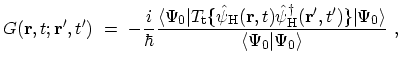(3.2)

where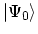is the ground-state of the interacting system in the HEISENBERG picture (Appendix B) and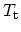is the time-ordering operator defined in (B.21). The field operator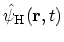in the HEISENBERG picture is given by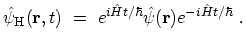(3.3)

Inserting (3.3) into (3.2), the physical interpretation of the GREEN's function becomes obvious(3.4)

If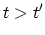, the GREEN's function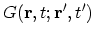is the probability amplitude that a particle created at time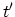at place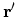moves to time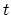and place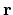. This follows from the definition of. At zero time the system is at the ground-state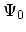. The system then evolves to timewith the operator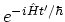. At this time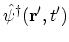creates a particle at place. Then, the system continues its evolution fromtowith the operator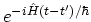, after which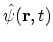annihilates the particle at place. The system returns to the initial ground-state with the operator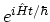. In a similar way, if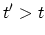, the field operator creates a hole at time, and the system then propagates according to the HAMILTONian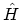. These holes can be interpreted as particles traveling backward in time . The probability amplitude that a hole created at timeat placemoves to timeand placeis again just the GREEN's function for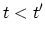.

To calculate, a perturbation expansion is very useful. However, the definition of the GREEN's function in (3.2) does not allow a direct solution, since it involves the exact ground-states of the interacting HAMILTONian, which is one of the things to be calculated. In the interaction representation the HAMILTON ian is expressed in terms of the non-interacting and interacting parts, see the equation (3.1). The ground state of the non-interacting part,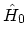, can be calculated easily. Therefore, one tries to express the ground state of the interacting systemin terms of the ground state of the non-interacting one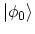. For that purpose, in equation (B.18) one adds to the operator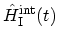a factor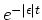, which switches the interaction off at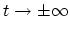. The non-interacting ground stateis assigned to the system at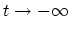and the connection tois formed by the GELL-MANN and Low theorem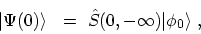(3.5)

where the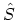operator in defined in Appendix B.4. The traditional argument is that one starts fromwith a wave function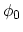which does not contain the effects of the interaction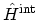. The operator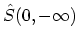brings this wave function up to the present,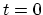. Thus one has the wave function which contains the effects of the interaction, so that it is an eigenstate of. As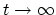, one gets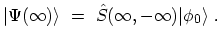(3.6)

One possible assumption is that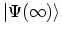must be related to. The system returns to its ground state forexcept for a phase factor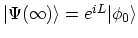which implies that,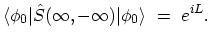(3.7)

An alternative to this assumption is discussed in Section 3.3.2.

Using the relation (3.5) for the ground-state, equation (3.2) becomes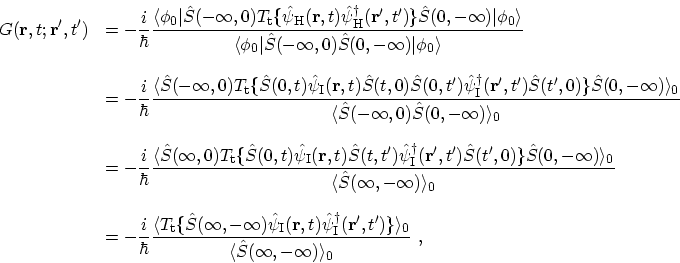(3.8)

where the short-hand notation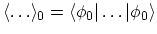is introduced to represent the expectation value over the ground-state of the non-interacting system at zero temperature. The transition from the first to the second line is achieved by using (B.13) for converting the HEISENBERG representation of operators into the interaction representation. The second step is obtained by taking account of the properties of theoperators described in Appendix B.4 and the return of the system to its ground-state as. In the forth line the operator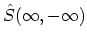contains several time intervals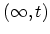,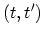, and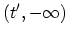. Theoperator automatically sorts these intervals so that they act in their proper sequences. Replacing operatorwith its formal definition (see (B.24)) one gets(3.9)

M. Pourfath: Numerical Study of Quantum Transport in Carbon Nanotube-Based Transistors